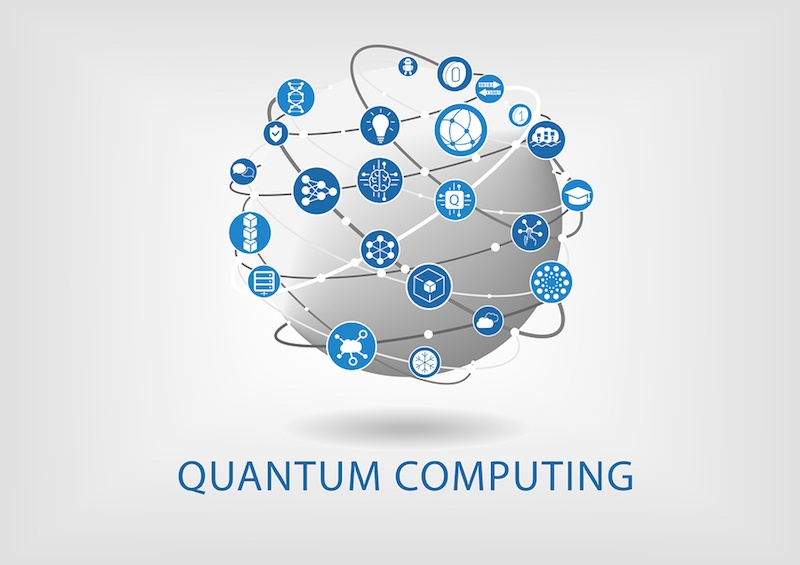Quantum computing is a paradigm of computing different from the one that rules classical computing. It is based on the use of qubits instead of bits and gives rise to new logical gates that make possible new algorithms.

## The basic states of qbit: the amount of information

A qubit is a quantum system with two eigenstates that we can manipulate arbitrarily. The qubit is the minimum unit and from the theory of quantum information is created based on it. The qbit is a fundamental concept for quantum computing. In fact, it is the equivalent to the bit in classical computing.
The two basic states of a qubit are 0 and 1, just the same as the classic bit. But in addition, the qubit can be in a state of quantum superposition that is a combination of those two states. This is significantly different from the state of a classic bit. As it can only have the values 0 or 1.
Its importance lies in the fact that the amount of information contained in a qubit, and the way we can manipulate this information is completely different from a classical bit. There are logical operations that are possible in a qubit but not in a bit.
The same task may have different complexity in classical computing and quantum computing. This has led to great expectation since some intractable problems become treatable.

## The qbits and how they overcome physical limitations of processing

As technology evolves, the scale of integration increases and more transistors fit in the same space. Microchips are thus made smaller and smaller. The smaller a processor is, the faster the process reaches the chip. However, we can not make infinitely small chips. There is a limit at which they stop working correctly. When we reach the nanometer scale, the electrons escape from the channels through which they must circulate. This is called the tunnel effect.
If a classic particle encounters an obstacle, it can not pass through and bounces. But with electrons, which are quantum particles and behave like waves, things change. There is a possibility that a part of them could pass through the walls if they are thin enough. Thus, the signal can pass through channels where it should not circulate. Therefore, the chip stops working correctly.
It is at this point when the need to discover new technologies arises and where quantum computing enters the scene.

## How quantum computing works

The idea of quantum computing arose in 1981, when Paul Benioff presented his theory to take advantage of quantum laws in the computing environment. Instead of working at the level of electrical voltages, it works at the quantum level. In digital computing, a bit can only take two values: 0 or 1. In contrast, in quantum computing, the laws of quantum mechanics intervene. So the particle can be in a coherent superposition: it can be 0, 1 and it can be 0 and 1 at the same time. This allows several operations to be carried out at once.
If you are interested in new technologies and how the digital world develops, keep reading our blog!